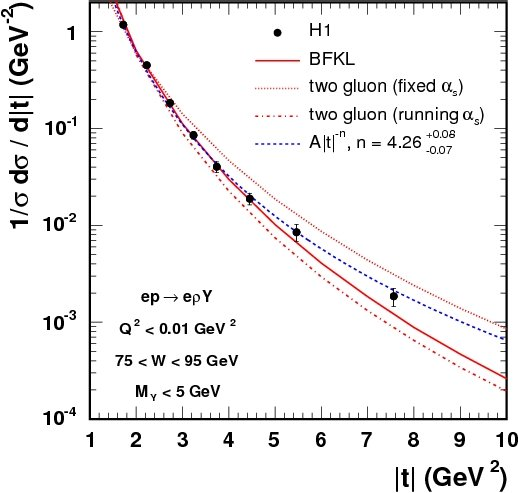# The H1 Collaboration

 Results are presented of the diffractive production of rho meson in ep interactions, e p -> e rho Y ( rho -> pi+ pi-), in the case where the negative four momentum transfer squared at the proton vertex, t, is large. Here, the system Y represents either an elastically scattered proton or a low mass dissociated system of mass MY < 5 GeV. The data, corresponding to an integrated luminosity of 20 pb-1, are taken with the H1 detector in the kinematic range Q2 < 0.01 GeV2, 75 < W < 95 GeV and 1.5 < |t| < 10 GeV2, where Q2 is the modulus squared of the four-momentum carried by the intermediate photon, W is the gamma-p centre of mass energy. The large momentum transfer provides the hard scale necessary for the application of perturbative QCD (pQCD) models. The results are compared to two theoretical predictions: a fixed order calculation in which the hard interaction is approximated by the exchange of two gluons and a LL calculation in which it is described according to the BFKL evolution. The t dependence of the e p -> e rho Y cross section is presented in Fig. 1. Both the experimental result and the theoretical predictions are normalised to unity. Over the measured t range, the data are well described by the BFKL model (solid line) in contrast to predictions of the two-gluon model with fixed (dotted line) or running (dashed-dotted line) alpha_S. The spin density matrix elements characterise the helicity states of the rho meson and the intermediate photon. The Fig. 2 presents the dependences versus |t| of the r0400, Re[r0410] and r041-1 matrix elements extracted from the H1 data together with previous results from the ZEUS Collaboration. The small values of r0400 indicate that the probability of producing a longitudinally polarised rho meson from a transversely polarised photon is low. The non-zero values of Re[r0410] and r041-1 indicate however a violation of s-channel helicity conservation (SCHC) between the intermediate photon and the produced rho meson. The two-gluon predictions (dotted lines) are unable to describe the measured spin density matrix elements. For the BFKL predictions (solid lines), r0400 is well described but the prediction for r041-1 is too negative and the wrong sign for Re[r0410] is predicted. Although, unlike the two-gluon models, the BFKL model is able to describe a predominantly longitudinal rho meson production, both considered models fail to describe the full set of spin density matrix elements.Fig. 1: The t dependence of the e p -> e rho Y cross section. Fig. 2: Some spin density matrix elements for rho meson production versus t.